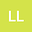Blowup of sign-changing radial solutions for a semilinear parabolic equation
•• Linfeng Luo
Linfeng Luo
Xi'an Jiaotong University

Corresponding Author:[email protected]

Author Profile## Abstract

This paper is concerned with sign-changing radial solutions of the semilinear parabolic equation $$\left\{ \begin{gathered} u_t-u_{rr}-\frac{N-1}{r}u_r=a(r)u+|u|^{p - 1}u, \ \ \ \ r\in(0,1), t>0, \hfill \\ u_r(0,t)=0, \ \ \ \ \ \ \ \ \ \ \ \ u(1,t)=0, \ \ \ \ \ \ \ \ \ \ \ \ t>0 \hfill \\ \end{gathered} \right.\leqno{\rm({P})}$$ with initial data $u(r,0)=u_0(r)$, $r\in[0,1]$, where $u_0(r)$, $a(r)\in C[0,1]$, $u_0(r)$ is not identically equal to $0$ in $[0,1]$, $p>1$, $N>1$. Under suitable assumptions on $\lambda_k$, we prove that solutions blowup in finite time if $z(u_0)\leq k$, while there exist stationary solutions with $k$ or more zeros, where $\lambda_k$ is the k-th eigenvalue of linearized equation, and $z(\cdot)$ is the number of times of sign changes.